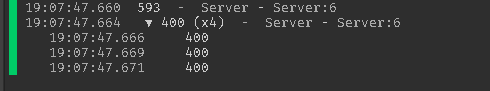# How can i randomize something on a for loop?

1. What do you want to achieve?
Making a randomized spawn

To make this simple i have a module script for spawns, But to make this simplier ill just be be using math.random for this example. The first run through the loop is perfectly fine, while the rest is all the same. Example: 5 (1st random) 10 (2nd) 10 (3rd) 10 (4th)``````for i=1,5 do
print(math.random(1,600))
end
``````

I already have a script for it. Do all spawnpoints have the same Y level? (up & down)

Yes they are on the same Y level.

Then simply do this:

``````local x = math.random(-500,500)
local y = (The specific Y level)
local z = math.random(-500,500)
-- Those can be any values, I put mine so the region is from -500,-500 to 500 500
local clone = character.Clone:()
clone.Parent = Workspace
clone:MoveTo(Vector3.new(x,y,z))
``````

If its a player you want to spawn randomly, do this:

``````local x = math.random(-500,500)
local y = (The specific Y level)
local z = math.random(-500,500)
– Those can be any values, I put mine so the region is from -500,-500 to 500 500
player.Character:MoveTo(Vector3.new(x,y,z))
``````

I wasn’t looking for this sorry but thank you! I wanna understand why its just constantly printing the same number after it runs through the for loop one time? I’m using randomized spawns by using parts, I have everything setup! I just don’t understand why it wont rerandomize it self after it runs?

Oh sorry! Let me check by myself

Fixed! Since math.random uses pseudorandom number generation, i needed to add a wait! Sorry for the hassle anyone reading this.

Oh no worries!
I just ran it on my studio and it worked so I was quite confused xD

1 Like

If you don’t want to busy wait you can replace the yield with `math.randomseed(os.clock())`.

1 Like